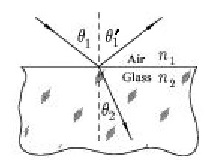# Problem: True or False. The light ray that makes the angle θ1' is the incident ray.

###### FREE Expert Solution

Snell's law:

$\overline{){{\mathbf{\eta }}}_{{\mathbf{1}}}{\mathbf{s}}{\mathbf{i}}{\mathbf{n}}{{\mathbf{\theta }}}_{{\mathbf{1}}}{\mathbf{=}}{{\mathbf{\eta }}}_{{\mathbf{2}}}{\mathbf{s}}{\mathbf{i}}{\mathbf{n}}{{\mathbf{\theta }}}_{{\mathbf{2}}}}$

The incident light upon striking a surface is split into the reflected ray and a transmitted ray.

The angle between the reflected ray and the normal and the angle between the incident ray and the normal are equal according to the the law of reflection.

87% (317 ratings)###### Problem Details

True or False. The light ray that makes the angle θ1' is the incident ray.